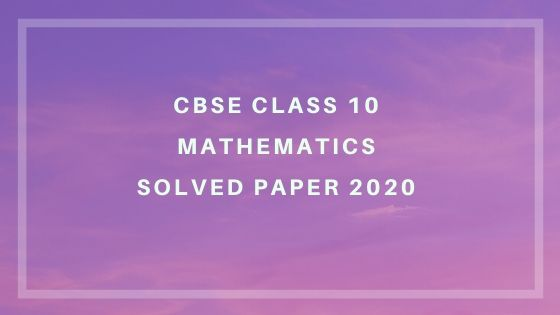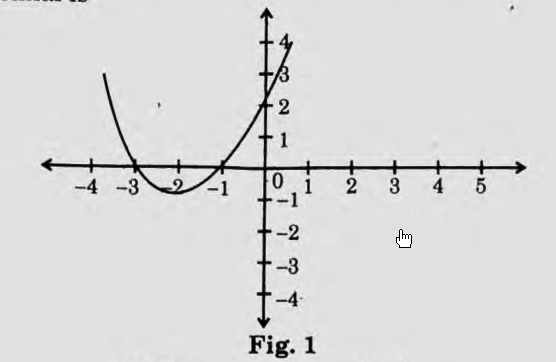# CBSE 10th Maths Exam 2020 Question Paper and Solutions

### Question Paper and Answer key of CBSE Class 10 Mathematics (Standard) ExamQuestion Paper and Answer key of the CBSE Class 10 Mathematics (Standard) Examination held on 13th March 2020. The question paper code is 30/3/2\

## CBSE Class 10 Maths Question Paper and Answer Keys 2020

### Section – A

Question number 1 to 10 are multiple choice questions of 1 mark each. You have to select the correct choice :

1 In fig 1. the graph of the polynomial p(x) is given. The number of zeroes of the polynomial is(a) 1
(b) 2
(c) 3
(d) 0

Ans: (b)

2. If (a, b) is the mid-point of the line segment joining the point A(10, -6) and B(k, 4)and a-2b=18, the value of k is
(a) 30
(b) 22
(c)4
(d) 40

Ans: (b)

3. The value of k for which the points A (0, 1), B (2, k) and C(4, -5) are collinear is
(a) 2
(b) -2
(c) 0
(d) 4

Ans: (b)

4. If ∆ABC ~ ∆DEF such that AB = 1.2 cm and DE = 1.4 cm, the ratio of the areas of ∆ABC and ∆DEF is
(a) 49:36
(b) 6:7
(c) 7:6
(d) 36:49

Ans: (d)

5. The HCF of 135 and 225 is
(a) 15
(b) 75
(c) 45
(d) 5

Ans: (c)

6. The exponent of 2 in the prime factorization of 144, is
(a) 2
(b) 4
(c) 1
(d) 6

Ans: (b)

7. The common difference of an AP, whose nth term is an=3n+7 is
(a) 3
(b) 7
(c) 10
(d) 6

Ans: (a)

8. The value of λ for which (x2+4x+ λ) is a perfect square, is
(a) 16
(b) 9
(c) 1
(d) 4

Ans: (d)

9.  The value of k, for which the pair of linear equations kx + y = k2 and x + ky = 1 have infinitely many solutions is
(a) ±1
(b) 1
(c)-1
(d) 2

Ans: (b)

10. The value of p for which (2p + 1), 10 and (5p + 5) are three consecutive terms of an AP is
(a) -1
(b) -2
(c) 1
(d) 2

Ans: (d)

OR

The number of terms of an AP 5, 9, 13, .. .. 185 is
(a) 31
(b) 51
(c) 41
(d) 40
Ans: Option not present, answer is 44

(Answer Key Prepared by Mrs. Leji Santhosh)

Download the detailed solutions of CBSE 10th March Exam 2020 Section B in PDF format from

CBSE 10th Exam 2020 Question Papers and Answer Keys-MATHS

### Related Pages:

•Muskan says:

I want solutions of standard set 3

•Shalini says:

Are jnv syudent

•Hariharan says:

Which language are you

•Hariharan says:

Ok

•Bts army says:

Can I get answers of maths set3 of code 30/5/3

•Nishant says:

Me also

•mohammed ashiq says:

r u thrayamika?

•Amrit raj says:

I also need the same….

•Sakshi says:

Me also

•Mouni says:

I did not get this paper.i want mathematics standard set 2 solutions

•Mohit says:

uska solution tujhe kl dunga

•Nandana says:

•Neha eliza binoy says:

section A is difficult and also 10th in section A has mistake in its option .pls you must give mark for that

•Mohit says:

Agar aise khne te no. Aate to paper me kch mt likh ke aati

•Sweta Sinha says:

Where we get 2020 cbse math question wid solution?

•netrimedia says:
•Anjana says:

This not good. Only section A questions. It’s very poor. I need complete soved answers

•Nandana says:

Yes

•Thowfiq says:

Me too